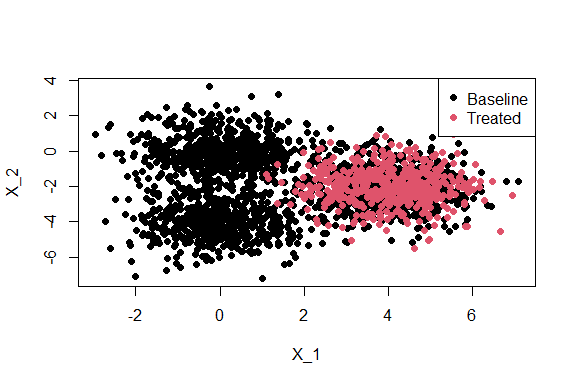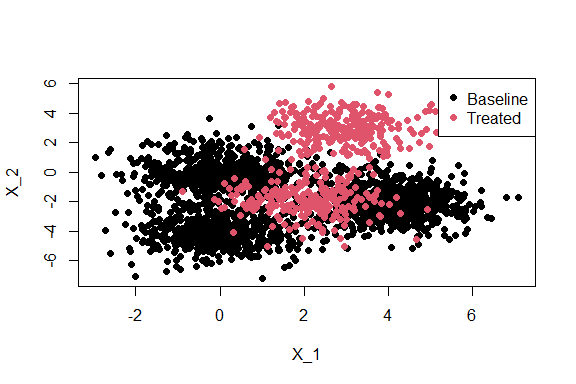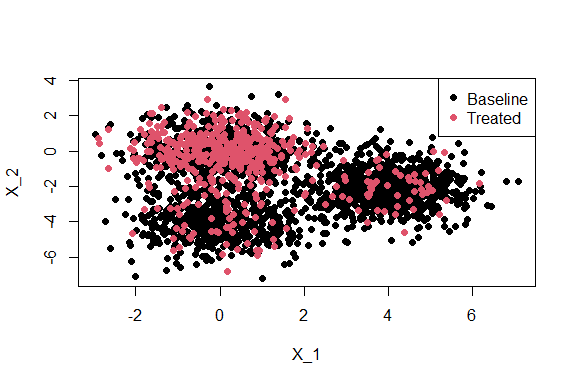# demo-truh

This vignette provides a quick demo of the truh package. The example that we consider here is taken from Figure 3 of the paper: Trambak Banerjee, Bhaswar B. Bhattacharya, Gourab Mukherjee Ann. Appl. Stat. 14(4): 1777-1805 (December 2020) <DOI: 10.1214/20-AOAS1362>.

We will consider a nonparametric two sample testing problem where the $$d$$ dimensional baseline (or uninfected) sample $$\boldsymbol{U}=(U_1,\ldots,U_n)$$ are i.i.d with cdf $$F_0$$ and the $$d$$ dimensional treated (infected) sample $$\boldsymbol{V}=V_1,\ldots,V_m$$ are i.i.d with cdf $$G$$. Here, we assume that the heterogeneity in the baseline population is reflected by $$K$$ different subgroups, each having unimodal distributions with distinct modes and cdfs $$F_1,\ldots,F_K$$, and mixing proportions $$w_1,\ldots,w_K$$ such that $F_0=\sum_{a=1}^{K}w_aF_a~\text{where}~w_a\in(0,1)~\text{and}~\sum_{a=1}^{K}w_a=1.$

The goal is to test the following composite hypothesis: $H_0:G\in\mathcal{F}(F_0)~\text{versus}~H_1:G\notin\mathcal{F}(F_0),$ where $$\mathcal{F}(F_0)$$ is the convex hull of $$F_1,\ldots,F_K$$. We take $$d=2,n=2000,m=500$$ and sample $$U_1,\ldots,U_n$$ from $$F_0$$ where $F_0=0.3N(\boldsymbol{0},\boldsymbol{I}_2)+0.3N(\boldsymbol{\mu}_1,\boldsymbol{I}_2)+0.4N(\boldsymbol{\mu}_2,\boldsymbol{I}_2),$ with $$\boldsymbol{\mu}_1=(0,-4)$$ and $$\boldsymbol{\mu}_2=(4,-2)$$.

n = 2000
d = 2

#Sampling the baseline (uninfected)
set.seed(1)
p<-runif(n,0,1)
set.seed(10)
U<- (p<=0.3)*matrix(rnorm(d*n),n,d)+
(p>0.3 & p<=0.6)*cbind(matrix(rnorm(n),n,1),
matrix(rnorm(n,-4),n,1))+
(p>0.6)*cbind(matrix(rnorm(n,4),n,1),
matrix(rnorm(n,-2),n,1))

To sample $$V_1,\ldots,V_m$$ we consider three settings for $$G$$.

• Setting 1: $$G=N(\boldsymbol{\mu}_2,\boldsymbol{I}_2)$$ which is the third component cdf of $$F_0$$. In this setting clearly $$G\in\mathcal{F}(F_0)$$ and the null hypothesis $$H_0$$ is true.
# Sampling the treated (infected)
m = 500
set.seed(50)
V1<-cbind(matrix(rnorm(m,4),m,1),
matrix(rnorm(m,-2),m,1))

#Scatter plot of the data
grp = c(rep('Baseline',n),
rep('Treated',m))
plot(c(U[,1],V1[,1]), c(U[,2],V1[,2]),
pch = 19,
col = factor(grp),
xlab = 'X_1',
ylab = 'X_2')

# Legend
legend("topright",
legend = levels(factor(grp)),
pch = 19,
col = factor(levels(factor(grp))))• Setting 2: $$G=0.5N(\boldsymbol{\mu}_3,\boldsymbol{I}_2)+0.5N(\boldsymbol{\mu}_4,\boldsymbol{I}_2)$$ where $$\boldsymbol{\mu}_3=0.25\boldsymbol{\mu}_1+0.5\boldsymbol{\mu}_2$$ and $$\boldsymbol{\mu}_4=(3/4)\boldsymbol{\mu}_1+(9/8)\boldsymbol{\mu}_2$$. Clearly in this case $$G\notin\mathcal{F}(F_0)$$.
# Sampling the treated (infected)
m = 500
set.seed(20)
q<-runif(m,0,1)
set.seed(50)
V2<-(q<=0.5)*cbind(matrix(rnorm(m,2),m,1),
matrix(rnorm(m,-2),m,1))+
(q>0.5)*cbind(matrix(rnorm(m,3),m,1),
matrix(rnorm(m,3),m,1))

#Scatter plot of the data
plot(c(U[,1],V2[,1]), c(U[,2],V2[,2]),
pch = 19,
col = factor(grp),
xlab = 'X_1',
ylab = 'X_2')

# Legend
legend("topright",
legend = levels(factor(grp)),
pch = 19,
col = factor(levels(factor(grp))))• Setting 3: $$G=0.8N(\boldsymbol{0},\boldsymbol{I}_2)+0.1N(\boldsymbol{\mu}_1,\boldsymbol{I}_2)+0.1N(\boldsymbol{\mu}_2,\boldsymbol{I}_2)$$. This is the most interesting setting as here $$G\in\mathcal{F}(F_0)$$ but $$G\neq F_0$$ because the mixing weights differ.
# Sampling the treated (infected)
m = 500
set.seed(20)
q<-runif(m,0,1)
set.seed(50)
V3<-(q<=0.8)*matrix(rnorm(d*m),m,d)+
(q>0.8 & q<=0.9)*cbind(matrix(rnorm(m),m,1),
matrix(rnorm(m,-4),m,1))+
(q>0.9)*cbind(matrix(rnorm(m,4),m,1),
matrix(rnorm(m,-2),m,1))

#Scatter plot of the data
plot(c(U[,1],V3[,1]), c(U[,2],V3[,2]),
pch = 19,
col = factor(grp),
xlab = 'X_1',
ylab = 'X_2')

# Legend
legend("topright",
legend = levels(factor(grp)),
pch = 19,
col = factor(levels(factor(grp))))Let us now execute the truh testing procedure for these scenarios. Recall that the goal is to test the following composite hypothesis: $H_0:G\in\mathcal{F}(F_0)~\text{versus}~H_1:G\notin\mathcal{F}(F_0).$ - Setting 1: Here we know that $$G=F_0$$ and so $$H_0$$ is true.

library(truh)
truh.1 = truh(V1,U,B=200)
truh.1$pval ##  0.375 So, truh fails to reject the null hypothesis. • Setting 2: Here we know that $$G\notin\mathcal{F}(F_0)$$ and so $$H_0$$ is false. library(truh) truh.2 = truh(V2,U,B=200) truh.2$pval
##  0

We see that truh rejects the null hypothesis.

• Setting 3: Here $$G\in\mathcal{F}(F_0)$$ but $$G\neq F_0$$. The null hypothesis $$H_0$$ is true in this setting.
library(truh)
truh.3 = truh(V3,U,B=200)
truh.3\$pval
##  0.205

In this case, truh makes the correct decision and fails to reject $$H_0$$.